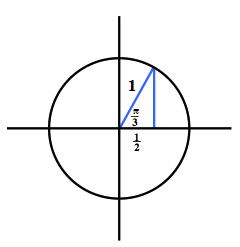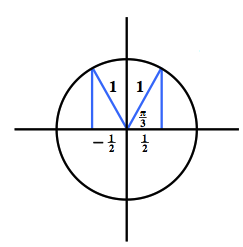### Home > INT3 > Chapter 10 > Lesson 10.2.1 > Problem10-107

10-107.

Use reference angles, the unit circle, and the fact that $\text{cos}(\frac{\pi}{3})=\frac{1}{2}$ to write three other true statements using cosine and angles that are multiples of $\frac { \pi } { 3 }$.

Draw a unit circle diagram for the given angle.Unit circle, with right triangle in first quadrant, labeled as follows: hypotenuse, 1, horizontal leg, 1 half, angle opposite vertical leg, pi thirds.

Reflect the triangle in each of the 4 quadrants. Quadrant 2 is shown for you. Added to the unit circle, a right triangle in second quadrant, labeled as follows: hypotenuse, 1, horizontal leg, negative 1 half.

$\text{cos}\left(\frac{2\pi}{3}\right)=-\frac{1}{2}$